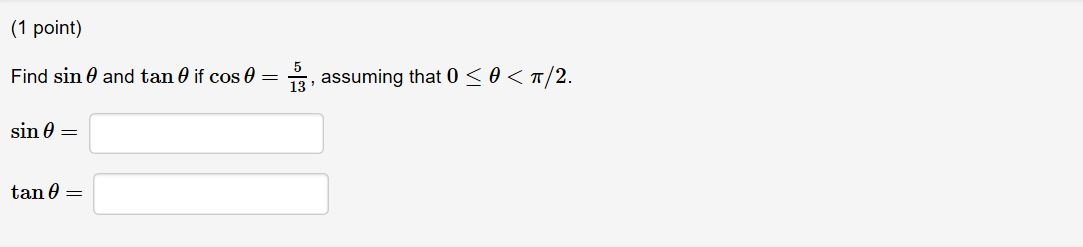(1 point)Find sin θ and tan θ if cos θsin θ-13, assuming that 0 < θ < π/2.tan θ

Question

Help.help_outlineImage Transcriptionclose(1 point) Find sin θ and tan θ if cos θ sin θ -13, assuming that 0 < θ < π/2. tan θ fullscreen
Step 1

Given:

The known value,

Step 2

Formula used:

Step 3

To find the opposite side of the right angle triangl...

Want to see the full answer?

See Solution

Want to see this answer and more?

Our solutions are written by experts, many with advanced degrees, and available 24/7

See Solution
Tagged in

Calculus